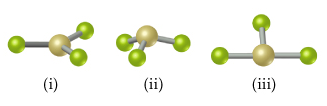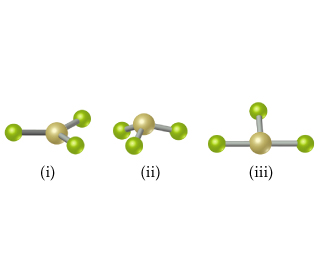# Problem: This figure shows ball-and-stick drawings of three possible shapes of an AF3 molecule. For each shape, give the electron-domain geometry on which the molecular geometry is based.

###### FREE Expert Solution

We’re being asked to determine the electron-domain geometry on which the molecular geometry of the ball-and-stick drawings of the three possible shapes of an AF3 molecule shown below:The possible electron pair and molecular geometries are:Looking at the chart, the molecular geometry and electron-domain geometry of the 3 structures of AF3 are the following:

79% (378 ratings)###### Problem Details

This figure shows ball-and-stick drawings of three possible shapes of an AF3 molecule.For each shape, give the electron-domain geometry on which the molecular geometry is based.